Function Repository Resource:

# RandomComposite

Find a random composite number or set of random composite numbers

Contributed by: Peter Cullen Burbery
 ResourceFunction["RandomComposite"][n] returns a random composite number up to n. ResourceFunction["RandomComposite"][n,m] returns m random composite numbers up to n. ResourceFunction["RandomComposite"][{min,max}] returns a random composite number between min and max. ResourceFunction["RandomComposite"][n, arrayspec] returns an array of random composite numbers up to n.

## Details

RandomComposite can return duplicates.

## Examples

### Basic Examples (2)

Find a random composite number less than or equal to the a ceiling of the exponential of an integer:

 In:=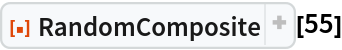Out=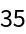Find a random composite number between 149 and 404:

 In:=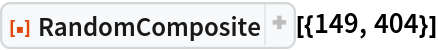Out=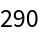### Scope (5)

Find a list of 30 random composite numbers between 1097 and 2981:

 In:=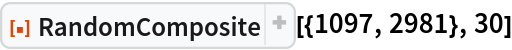Out=Find a 3⨯3 matrix of random composite numbers between 8104 and 22027 and print it in matrix form:

 In:=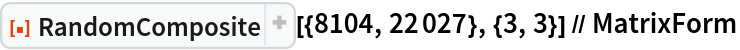Out=Find a 3⨯3⨯3 array of random composite numbers between 59875 and 162755 and print it in matrix form:

 In:=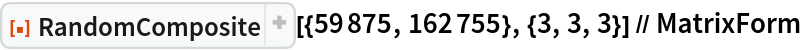Out=Find a 3⨯3⨯3⨯3 tensor of random composite numbers between 442414 and 1202605:

 In:=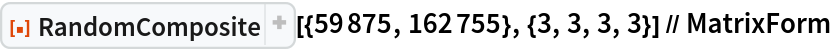Out=Find a large random composite up to a googol:

 In:=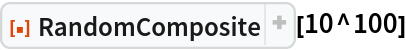Out=### Possible Issues (2)

The function can return duplicates:

 In:=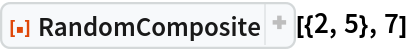Out=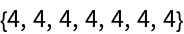This is similar to the behavior of RandomPrime, which also produces duplicates:

 In:=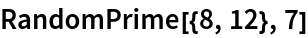Out=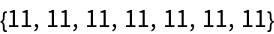Peter Burbery

## Requirements

Wolfram Language 13.0 (December 2021) or above

## Version History

• 1.0.1 – 28 July 2023
• 1.0.0 – 06 June 2023

## Author Notes

There was a bug where the function would only work if the min was greater than or equal to 4. For example, neither RandomComposite[{2,5}] nor RandomComposite[{3,5}] would work. Now I have fixed it so it works as long as the min is greater than 0.